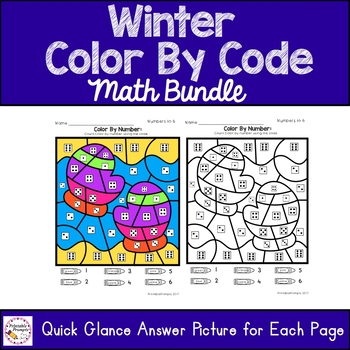DID YOU KNOW:
Seamlessly assign resources as digital activities

Learn how in 5 minutes with a tutorial resource. Try it Now

Learn More# Winter Color By Number Math BundleK - 2nd
Subjects
Standards
Resource Type
Formats Included
• Zip
Pages
48 pages
\$4.80
Bundle
List Price:
\$6.00
You Save:
\$1.20
\$4.80
Bundle
List Price:
\$6.00
You Save:
\$1.20

### Description

Engage your students in winter themed color by number and addition practice with easy differentiation. This resource includes the fall themed color by number, color by sum with addition to 10, and addition to 20 which both include doubles facts as well. The 8 winter and snow themed pictures are the same in each resource, but the content on each picture changes, so you could have 3 different groups or students working at the level that best suites them! These are great for math centers, substitute plans, math fact homework, early finishers, or morning math tubs in December, January, February or even the beginning of March!

Includes:

*8 color by number pages (color by dice dot pattern and numbers to 10) along with a quick glance answer picture for each

*8 color by sum for addition to 10 and doubles to 12 (using equations and dice dot patterns)

*8 Color by sum addition to 20 (doubles and mixed fact practice)

Total Pages
48 pages
Included
Teaching Duration
N/A
Report this Resource to TpT
Reported resources will be reviewed by our team. Report this resource to let us know if this resource violates TpT’s content guidelines.

### Standards

to see state-specific standards (only available in the US).
Fluently add and subtract within 20 using mental strategies. By end of Grade 2, know from memory all sums of two one-digit numbers.
Add and subtract within 20, demonstrating fluency for addition and subtraction within 10. Use strategies such as counting on; making ten (e.g., 8 + 6 = 8 + 2 + 4 = 10 + 4 = 14); decomposing a number leading to a ten (e.g., 13 - 4 = 13 - 3 - 1 = 10 - 1 = 9); using the relationship between addition and subtraction (e.g., knowing that 8 + 4 = 12, one knows 12 - 8 = 4); and creating equivalent but easier or known sums (e.g., adding 6 + 7 by creating the known equivalent 6 + 6 + 1 = 12 + 1 = 13).
Fluently add and subtract within 5.
Understand the relationship between numbers and quantities; connect counting to cardinality.
Write numbers from 0 to 20. Represent a number of objects with a written numeral 0-20 (with 0 representing a count of no objects).